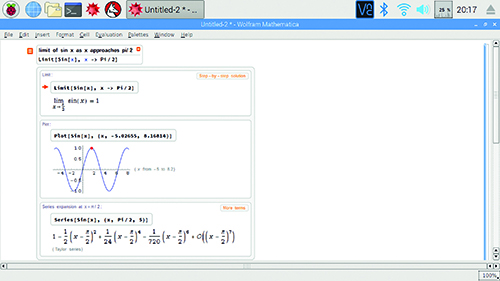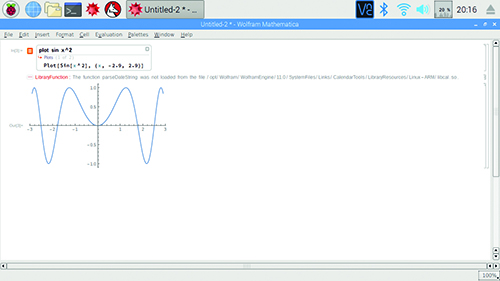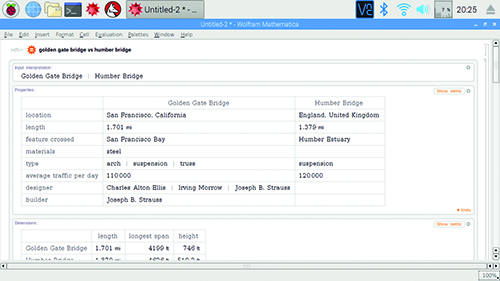# Use Wolfram Mathematica on Raspberry Pi

By Lucy Hattersley. Posted

Many professionals who work with numbers pay hundreds of pounds to purchase Mathematica. It’s an extremely powerful mathematics and data processing program. Normally it costs hundreds of pounds to buy a licence, but Mathematica is free on the Raspberry Pi.

Back in issue 67 we looked at Wolfram Language. This programming syntax can be used in Mathematica, where its graphical interface provides detailed graphs, plots, and further information.

Anybody who is learning maths or works with numbers will value Mathematica.
Click on the Mathematica icon in the Task Bar to start the program, or choose Menu > Programming > Mathematica.

The default entry is Wolfram Language but unlike the command-line environment, you run lines using SHIFT+ENTER. Type out:

`2+2`

And press SHIFT+ENTER to get the result.

`In:= 2+ 2`
` Out:= 4`

To the right of the screen next to each are vertical lines knows as ‘cell brackets’. There is one for the input and output, and a larger one grouping both the input and output. You can highlight, cut, and copy cells with these.### Using Wolfram Mathematica on Raspberry Pi

In Mathematica you can enter fractions by pressing CTRL+/. This splits the input into a numerator and denominator (indicated by empty boxes). Use your arrow keys and SHIFT to highlight the boxes and enter the amounts, such as:

`        2   3`
` In[n]:= - * -`
`        4   5`

`3`
` Out[n]= -`
`       10`

There are lots of other techniques for entering math symbols. Such as CTRL+2 to create and insert a square root, and pressing ESC, P, ESC to enter the symbol (π) for pi.

Rather than memorise them all, it’s better to open Palettes > Basic Math Assistant. Here you’ll see an advanced calculator-style window, with buttons for math symbols and functions.

### Free-form input in Wolfram Mathematica

One of the more interesting advantages Mathematica has over Wolfram Language in the command line is free-form Input. This enables you to enter equations in plain English and get expanded information.

Press the equals key (=) once at the start of a new cell entry to switch to free-form input. Notice that the line now starts with an orange equals sign.

Before we go any further, press the = key a second time and the cursor will change again to an equals sign in a starburst shape. This is Wolfram|Alpha query, which we’ll come to in a bit. Press BACK SPACE to get back to free-form input.
In free-form input, you can enter regular expressions such as:

`2+2`

As well as the result (4), you’ll see a box appear around the input.
Look closely at the box and you’ll see a small plus (+) symbol. Hover the mouse to see ‘Show all results’ in a pop-up balloon.

Click this and you’ll see detailed information, such as the result, number line, manipulatives illustration, and even typical human computation times.

Now click on ‘Step-by-step solution’ and you’ll get a detailed breakdown, including a number line (which you can change to ‘math manipulatives’). It might seem silly for 2+2, but try it with something complex like these polynomials:

`= (5a2-6a-4) - (-7a2+3a-9)`

Now click ‘Show all results’ and ‘Step-by-step solution’ and you’ll get a full breakdown of the intermediate steps. Handy if you’re learning mathematics and want to check your own steps.

### Simple plotting with Wolfram Mathematica

Free-form input enables you to enter commands with the formality of Wolfram Language. Enter:

`plot sin x2`

In free-form input this is converted to the formal Wolfram Language command:

`Plot[Sin[X2, {x, -2.9, 2.9}]].`

You’ll get to see a nice graph of the result (see Figure 1). Click the ‘Show all results’ button to see a range of subpods with sliders and controls, enabling you to fully explore the mathematical concept.Free-form input is great for more advanced subjects, such as finding limits.

`limit of sin x as x approaches pi/2`

Click the ‘Show all results’ button to view the answer (1) as well as a plot of the graph with a red dot at the limit. Below it you’ll even get a series expansion.

### Wolfram|Alpha query

Beyond mathematics lies the wonderful world of the Wolfram|Alpha computational knowledge engine. This can also be used for advanced maths. However, its real strength is immediate access to a huge database of facts and stats. Want to know if more people live in London or New York?:

`population of London > New York`

`True`

Or get information on structures?:

`golden gate bridge vs humber bridge`Wolfram|Alpha displays a wealth of information such as the location, length, and average traffic per day. It even displays photographs and a Wikipedia summary (see Figure 2). All of this data could be accessed by Wolfram Language, but the format is quite complex.

There’s a lot of support from Wolfram. Mathematica is a very deep and powerful piece of software, and Wolfram has provided lots of resources and documentation, which you can access using Help > Online Documentation.## From The MagPi store